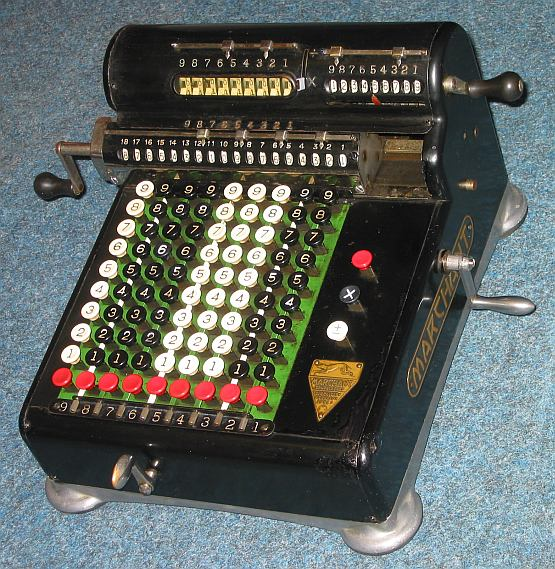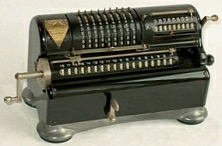previous <<==>> next

 MARCHANT  H9 Have a look at the MARCHANT »STANDARD« ...Have a look at the Restoration Procedure ...
 ``` T E C H N I C A L D A T A of the Mechanical Calculator MARCHANT H9 ****************************************************************************** Serial Number: H-9-6253 Dimensions: (ca.) Width = 13_1/2" / 34 cm Depth = 14 " / 35 cm Height = 7_1/2" / 18 cm Weight: (ca.) 24 lbs / 11 kg Mechanics: 9*10 Full-Keyboard Adapting Segment / StellSegment + Animation Functions: Add, Subtract, Multiply, Divide Registers: Input = 9 Decimals Counter = 9 Decimals Arithmetic = 18 Decimals Manufacturer: MARCHANT Calculating Machine Co. Oakland, California / USA 1925 R E M A R K : ************* Instead of "Full-KBD" also available with "Lever-Input" as Model "XL" ...H O W T O U S E the MARCHANT H9 **************************************** BEFORE STARTING A TASK: ----------------------- Turn the MAIN CRANK in its bottom (idle) position. CLEAR the KEYBOARD by pressing the right red key. ( The upper left DISPLAY UNIT shows the actual input 0...00 ) RELEASE the black REPEAT KEY (X) by pressing the right white (+/-) key. CLEAR the COUNTER unit by turning the upper right crank clockwise. CLEAR the ARITHMETIC unit by turning its left crank counter-clockwise. Bring the CARRIAGE to the far left ( = POSITION 1) either (a) by pulling and press & hold the BUTTON ON THE RIGHT SIDE - OR (b) step-by-step with the HANDLE in front ( The arrow on the counter moves right to show position 1 ) It depends of the UPPER CENTRAL LEVER how the COUNTER is operating: For ( low = X = default ) the counter will increase its content for positive (= Addition) turns and decrease for negative (= Subtraction) turns. In its ( up = / ) position the counter will operate contrary. In both cases the counter works with 10s-carry - allowing "Shortened Method of Multiplication". When using the MARCHANT H-9 for the 1st time, it seems strange that the arithmetic unit does not line up with the input unit ... REMARK to the 10s-Carry of the Arithmetic Unit in the MARCHANT H-9: ------------------------------------------------------------------- Because 10s-carry is processed together with the body underneath, there is NO 10s-CARRY left of position 13 when the carriage is in its leftmost position! ADDITION & SUBTRACTION: ----------------------- Example: 123 + 45 - 6 = 162 ADD: Enter the first number (123) in the far right columns. The upper left display unit will show this number. Make a positive (clockwise) turn with the main crank to transfer the number into arithmetic unit. The counting unit displays the figure 1. Enter the second number (45). Make a positive turn with the main crank to add the number. The arithmetic unit displays the intermediate sum (168) and the counting unit displays the figure 2. SUBTRACT: Enter the third number (6). Make a negative (counter-clockwise) turn with the main crank. The arithmetic unit displays the result (162) and the counting unit is decreased by 1. REMARK: NEGATIVE RESULTS are displayed in the arithmetic unit as the COMPLEMENT of the next higher 10, 100, 1000, ... Example: -12 = 99...9988 MULTIPLICATION: --------------- Example: 123 x 45 = 5535 Clear counter and arithmetic units. Press the right (X) REPEAT-Key. Enter the multiplicand (123) in the far right columns. The multiplicator (45) has two digits, so the arithmetic unit is moved to position 2 with the handle in front. Repeat making positive (clockwise) turns with the main crank, until the first figure of the multiplicator (4) will appear in the 2nd position of the counter unit. Move the arithmetic unit with the handle in front to position 1. Repeat making positive turns with the main crank, until the second figure of the multiplicator (5) appears in the 1st position of the counter unit. The multiplication is done: The multiplicand (123) stays in the keyboard and is also visible in the display unit, the multiplicator (45) is in the counter unit and the result (5535) is in the arithmetic unit. DIVISION: --------- Example: 22 : 7 = 3.14285714 Remainder 2 Division requires 3 steps: (A) To Set the Dividend into Arithmetic Unit: ----------------------------------------- For the maximum number of decimals, bring the arithmetic unit to the far right position. Enter the dividend (22) in the far right columns. Make a positive (clockwise) turn with the main crank to transfer into arithmetic unit. (B) To Set the Divisor in the Keyboard: ----------------------------------- Clear the keyboard. Clear the counter unit. Set the counter lever up to (/). Press the right REPEAT-Button (X). Enter the divisor (7) in the right column. (C) To Divide: ---------- Make negative (counter-clockwise) turns with the main crank until the arithmetic unit shows an "underflow". Make one positive (clockwise) turn with the main crank. Move the carriage with the handle in front to the next left position. Repeat this procedure until the required number of decimals are calculated... The result (3.14285714) is in the counter unit, and the remainder (2) is in the arithmetic unit. The divisor (7) stays in the keyboard ( and in the display unit ), therefore an additional decimal can be estimated ... ( 2, cause 2 x 7 = 14). impressum: ****************************************************************************** © C.HAMANN http://public.BHT-Berlin.de/hamann 12/31/10 ```